# CBSE Class 10 Science Magnetic Effects of Electric Current Assignment Set C

Read and download free pdf of CBSE Class 10 Science Magnetic Effects of Electric Current Assignment Set C. Get printable school Assignments for Class 10 Science. Standard 10 students should practise questions and answers given here for Chapter 13 Magnetic Effect Of Electric Current Science in Grade 10 which will help them to strengthen their understanding of all important topics. Students should also download free pdf of Printable Worksheets for Class 10 Science prepared as per the latest books and syllabus issued by NCERT, CBSE, KVS and do problems daily to score better marks in tests and examinations

## Assignment for Class 10 Science Chapter 13 Magnetic Effect Of Electric Current

Class 10 Science students should refer to the following printable assignment in Pdf for Chapter 13 Magnetic Effect Of Electric Current in standard 10. This test paper with questions and answers for Grade 10 Science will be very useful for exams and help you to score good marks

### Chapter 13 Magnetic Effect Of Electric Current Class 10 Science Assignment

Question : The device used for producing current is called
a. ammeter
b. galvanometer
c. generator
d. voltmeter
Explanation: Electric current is produced by electric generator which converts mechanical energy into electricity.

Question : What does the tangent at any point on magnetic field lines indicate?
a. direction of magnetic field
b. direction of the force
c. direction of current
d. direction of induced current
Explanation: Tangent indicates the direction of the magnetic field. It just an alternative used when compass is not available

Question : Potential difference between a live wire and a neutral wire is
a. 150 volt
b. 220 volt.
c. 210 volt
d. 200 volt
Explanation: In our country the potential difference between the live and neutral wire is 220 V and the frequency is 50 Hz.

Question :  What is meant by magnetic field?
Answer :   Magnetic field is a region around a magnetic material in which the force of magnetism acts.It is a vector quantity

Question : What is the function of a galvanometer in a circuit?
Answer : Galvanometer is an electromechanical instrument used to detect or indicate the presence of current by deflection in a circuit. It consists of a pointer which can move along a scale with zero marked at its centre and is attached to a moving coil.

Question : Why does a compass needle get deflected when brought near a bar magnet?
Answer : The needle of a compass is a small magnet. When a compass needle is brought near a bar magnet, its magnetic field lines interact with that of the bar magnet and therefore the compass needle gets deflected.

Question : A straight wire carrying electric current is moving out of a plane of paper and is perpendicular to it. What is the direction of the magnetic field?
Answer : Magnetic field lines will be concentric circles in the plane of the paper and in anti-clockwise direction which can be found out by applying Right hand thumb rule.

Question : What happens when an iron core is inserted into a current carrying solenoid?
Answer : When an iron core is inserted into a current carrying solenoid, strength of the magnetic field produced inside the solenoid increases and it forms an electromagnet.

Question :  In a domestic electric circuit, mention the potential difference between live wire and neutral wire and frequency of AC?
Answer :  Potential difference in between live wire and neutral wire domestic circuit is 220 volt frequency of AC is 50 Hz.

Question : Under what condition does a current carrying conductor kept in a magnetic field experience maximum force?
Answer : A current carrying conductor kept in a magnetic field experiences maximum force when direction of current is at right angles to the direction of the magnetic field according to Fleming’s left hand rule.

Question : What happens to the magnetic field lines due to a current-carrying conductor when the current is reversed?
Answer : When we reverse the direction of electric current flowing in the current-carrying conductor, then the direction of magnetic field lines produced by it are also reversed.

Question : When does an electric short circuit occur ?
Answer :  Electric short-circuit occurs when :
(a) live wire incidentally touches neutral or earth wire

(b) Insulation gets hardened by the excessive use.

(c) current passed through wire is more than its rating.

(d) insulation around the current carrying wires is weak.

Question : State the purpose for which the following rules are used
i. Right hand thumb rule
ii. Fleming's left hand rule
iii. Fleming's right hand rule
Answer :  i. The right-hand thumb rule is used to find the directions of the magnetic field around a current carrying straight conductor.
ii. Fleming's left-hand rule (motor rule) helps in understanding the direction of the magnetic force acting on a conductor.

iii. Fleming's right-hand rule (dynamo rule) helps in understanding the direction of induced current when a conductor moves in a magnetic field.

Question : Explain house hold electric circuits. What are their relative advantages?
Answer :   There are two system of circuits :
i. Tree system : Electricity supplied to us for domestic purposes is 220 V and A.C. The current alternates its polarity 50 times in 1s i.e., its frequency is 50 Hz., 15 A switches and 15 A sockets are used where heavy currents are to be drawn e.g., in hot air blower, motor, geyser etc. and 5 A switches, sockets are used for bulbs, tubes, radio, C.T.V., refrigerator fans etc. The main supply is delivered to houses using a three-core wiring called live, neutral and the earth. The live wire is red (or brown) in colour and brings current. It is dangerous to touch live wire with bare hands. Neutral is black (or light blue) coloured wire and is used as return wire.
The third wire, green (or yellow) in colour is earth wire. Earth may be taken from pole or a metal plate sunk deep in the earth. It is safety measure and does not in any way affect the supply. Earth is usually connected to the body of the electric appliances. If at any time, the live wire incidentally touches the body of the electric appliance, the earth wire sends the current from the body of the appliances to the earth. Each appliance has a separate switch connected across live wire. The neutral reaches the appliance directly. All the appliances are connected in parallel so that switching off one circuit does not affect the other section. The parallel circuit also ensures that each appliance is connected to same voltage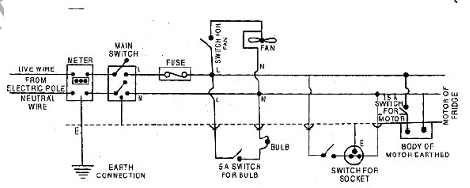a. Since all appliances are in parallel, each appliance gets optimum current.

b. Since all circuits are in parallel, P.D. at the ends of each appliance remains the same.

c. Each circuit is independent of the other. If there is a short-circuiting in one circuit then only the fuse in that circuit gets melted. Power supply to the other circuit remains unaffected.

d. Plugs and sockets to different amperage for different appliances are required.

e. Since all the circuits start from main distribution board, therefore, longer lengths of the wire are required for each circuit. It increases the cost of wiring system.

f. Installation is fairly time consuming

ii. Ring system of household wiring: Ring system consists of a ring-circuit starting distribution board and running around every corner and returns back to the distribution board again. There are two routes through which the current can flow through various appliances. Hence, a wire of lower rating may be used thus reducing the cost of installation.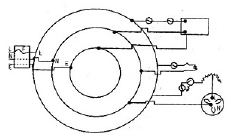a. All appliances have separate fuse. In case of short-circuiting, only one fuse goes away.

b. Its installation and maintenance is cheaper.

c. Since ring feeds whole house, the length of the wire used is much smaller. It is cost-saving.

ORAL QUESTIONS

Question : a) What are magnets?

b) What are natural magnets?

c) What is the meaning of the word lodestone?

d) What is the origin of the word magnetism?

Question : a) State the law of magnetic poles.

b) What is the surer test of magnetism?

c) What happens if we break a magnet into two pieces?

d) Is it possible to obtain isolated north and south poles?

Question : a) What is magnetic line of force?

b) Can two magnetic lines of force intersect? Give reason.

c) Magnetic lines of force are endless. Comment.

d) How do the field lines of the regions of strong field different from those of weak field?

Question : a) What is a solenoid?

b) Is the magnetic field of a solenoid similar to that of a bar magnet?

c) State the two factors by which the strength of magnetic field inside a solenoid can be increased.

d) How will you determine the direction of the magnetic field due to a current – carrying solenoid?

Question : a) What is an electromagnet?

b) What is the effect of placing an iron core in a solenoid?

c) What type of core should be used inside a solenoid to make an electromagnet?

d) Give two advantages of electromagnets.

ORAL QUESTIONS

Question : What important observation did Oersted make in his experiments with current carrying conductors?

Question : How can you locate a current – carrying wire concealed in a wall?

Question : A freely suspended magnet always points along north – south direction. Why?

Question : What type of core should be used inside a solenoid to make an electromagnet?

Question : Name the SI unit of magnetic field.

Question : What is the principle of an electric motor?

Question : A generator converts energy from one form to another. What is this energy conversion?

Question : Which wire (live, neutral or earth) goes through the switch?

Question : Are different appliances connected in series or parallel in a house?

Question : What is the colour convention for live, neutral and earth wires?

PUZZLE

• Across

1. A method preventing electric shock due to touching of live wire with the metallic body of an appliance.

2. A device to convert electrical energy into mechanical energy.

3. A device to convert mechanical energy into electrical energy.

4. SI unit of magnetic field.

• Down

5. A material having attractive and directive properties.

6. A temporary magnet.

The term magnetic effect of electric current means that an electric current flowing in a wire produces a magnetic field around it.
A current flowing in a wire always gives rise to a magnetic field around it. The magnetic effect of current is also called electromagnetism which means electricity produces magnetism. In figure, the deflection of compass needle by the current carrying wire in the below experiment show that an electric current produces a magnetic field around it.MAGNET
A magnet is an object, which attracts pieces of iron, steel, nickel and cobalt. It has two poles at ends – South and North Pole.
♦ Like magnetic poles repel each other.
♦ Unlike magnetic poles attract each other.

MAGNETIC FIELD
The space surrounding a magnet in which the force of attraction and repulsion is exerted is called a magnetic field.

MAGNETIC FIELD LINES
The magnetic field lines are the lines drawn in a magnetic field along which a north magnetic pole would move. These are also known as magnetic lines of forces.

PROPERTIES OF MAGNETIC FIELD LINES
1. A magnetic field lines originate from north pole and end at its south pole.
2. A magnetic field line is a closed and continuous curve.
3. The magnetic field lines are closer near the poles of a magnet where the magnetic field is strong and farther apart where the magnetic field is weak.
4. The magnetic field lines never intersect each other.
5. A uniform magnetic field is represented by parallel and equidistant field lines.INTEXT QUESTIONS PAGE NO. 224

1. Why does a compass needle get deflected when brought near a bar magnet?
Answer: A compass gets deflected due to the forces acting on its poles due to the magnetic field of the bar magnet.

MAGNETIC FIELD DUE TO A CURRENT THROUGH A STRAIGHT CONDUCTOR

The magnetic field lines around a straight conductor carrying current are concentric circles whose centres lies on the wire.
The magnitude of magnetic field produced by a straight current carrying wire at a point-
♦ directly proportional to current passing in the wire.
♦ inversely proportional to the distance of that point from the wire.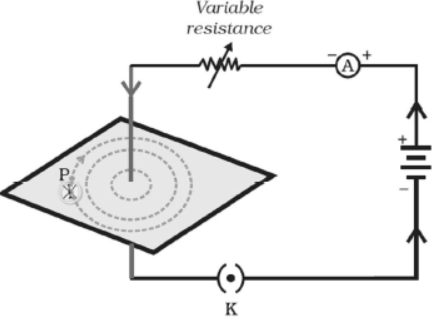RIGHT-HAND THUMB RULE
When a current-carrying straight conductor is holding in right hand such that the thumb points towards the direction of current. Then fingers will wrap around the conductor in the direction of the field lines of the magnetic field, as shown in below figure. This is known as the right-hand thumb rule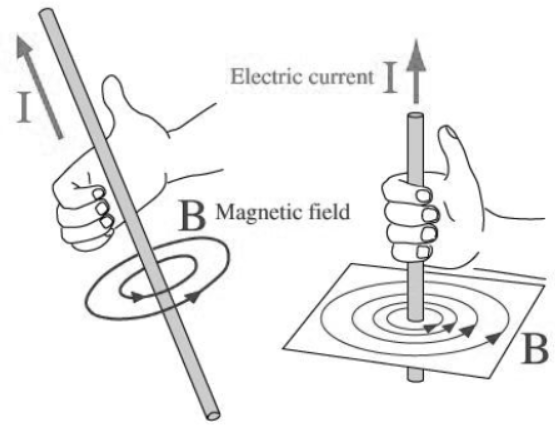Thumb-points in the direction of current then direction of fingers encircle the wire give the direction of magnetic field around the wire.

INTEXT QUESTIONS PAGE NO. 228

1. Draw magnetic field lines around a bar magnet.2. Why don’t two magnetic lines of force intersect each other?
Answer: If two magnetic lines of force intersect then there would be two directions of magnetic field at that point, which is absurd. That is why they never intersect.

MAGNETIC FIELD DUE TO A CURRENT THROUGH A CIRCULAR LOOP

The magnetic field lines are circular near the current carrying loop. As we move away, the concentric circles becomes bigger and bigger. At the centre, the lines are straight.
At the centre, all the magnetic field lines are in the same direction due to which the strength of magnetic field increase.
The magnetic of magnetic field produced by a current carrying circular loop at its centre is
♦ directly proportional to the current passing
♦ inversely proportional to the radius of the circular loop
The strength of magnetic field produced by a circular coil carrying current is directly proportional to both number of turns(n) and current(I) but inversely proportional to its radius(r).MAGNETIC FIELD DUE TO A CURRENT IN A SOLENOID

The insulated copper wire wound on a cylindrical tube such that its length is greater than its diameter is called a solenoid. The solenoid is from greek word for channel.
♦ The solenoid is a long coil containing a large number of close turns of insulated copper wire.
♦ The magnetic field produced by a current carrying solenoid is similar to the magnetic field produced by a bar magnet.
♦ The current in each turn of a current carrying solenoid flows in the same direction due to which the magnetic field produced by each turn of the solenoid ads up,
giving a strong magnetic field inside the solenoid.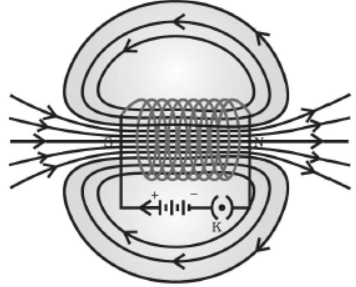The strong magnetic field produced inside a current-carrying solenoid can be used to magnetise a piece of magnetic material like soft iron, when placed inside the solenoid.
The magnet thus formed is called an electromagnet.
So, a solenoid is used for making electromagnets.
The strength of magnetic field produced by a carrying current solenoid depends on
♦ number of turns(n)
♦ strength of current(I)
♦ nature of core material used in solenoid – use of soft iron as core in a solenoid produces the strongest magnetism.

ELECTROMAGNETS AND PERMANENT MAGNETS
An electromagnet is a temporary strong magnet and is just a solenoid with its winding on soft iron core.
A permanent magnet is made from steel. As steel has more retentivity than iron, it does not lose its magnetism easily.

Difference between Electromagnet and permanent magnet

 Electromagnet Permanent magnet 1. An electromagnet is a temporary magnet as it can readily demagnetized by stopping the current through the solenoid. 1. A permanent magnet cannot be readily demagnetized. 2. Strength can be changed. 2. Strength cannot be changed. 3. It produces very strong magnetic forces. 3. It produces weal forces of attraction. 4. Polarity can be changed by changing the direction of the current. 4. Polarity is fixed and cannot be changed.

Q. Why soft iron is used for making the core of an electromagnet?
Answer: Soft iron is used for making the core of an electromagnet because soft iron loses all of its magnetism when current in the coil is switched off.

Q. Why steel is not used for making the core of an electromagnet?
Answer: Steel is not used for making the core of an electromagnet because steel does not loses all of its magnetism when current in the coil is switched off.

INTEXT QUESTIONS PAGE NO. 229 and 230

1. Consider a circular loop of wire lying in the plane of the table. Let the current pass through the loop clockwise. Apply the right-hand rule to find out the direction of the magnetic field inside and outside the loop.
For downward direction of current flowing in the circular loop, the direction of magnetic field lines will be as if they are emerging from the table outside the loop and merging in the table inside the loop. Similarly, for upward direction of current flowing in the circular loop, the direction of magnetic field lines will be as if they are emerging from the table outside the loop and merging in the table inside the loop, as shown in the given figure.2. The magnetic field in a given region is uniform. Draw a diagram to represent it.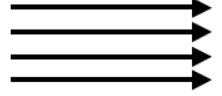3. Choose the correct option: The magnetic field inside a long straight solenoidcarrying current (a) is zero. (b) decreases as we move towards its end. (c) increases as we move towards its end. (d) is the same at all points.
The magnetic field for a point inside a long straight solenoid carrying current is double than for a point situated at one of its ends. Thus, the correct option is (b).

FORCE ON A CURRENT-CARRYING CONDUCTOR IN A MAGNETIC FIELD

When a current carrying conductor is placed in a magnetic field it experiences a force, except when it is placed parallel to the magnetic field.
The force acting on a current carrying conductor in a magnetic field is due to interaction between:
1. Magnetic force due to current-carrying conductor and
2. External magnetic field in which the conductor is placed.In the above figure, a current-carrying rod, AB, experiences a force perpendicular to its length and the magnetic field.
The displacement of the rod in the above activity suggests that a force is exerted on the current-carrying aluminium rod when it is placed in a magnetic field. It also suggests that the direction of force is also reversed when the direction of current through the conductor is reversed. Now change the direction of field to vertically downwards by interchanging the two poles of the magnet. It is once again observed that the direction of force acting on the current-carrying rod gets reversed. It shows that the direction of the force on the conductor depends upon the direction of current and the direction of the magnetic field. We considered the direction of the current and that of the magnetic field perpendicular to each other and found that the force is perpendicular to both of them.

FLEMING’S LEFT HAND RULE
Fleming's left hand rule (for electric motors) shows the direction of the thrust on a conductor carrying a current in a magnetic field. The left hand is held with the thumb,
index finger and middle finger mutually at right angles.
The First finger represents the direction of the magnetic Field. (north to south)
The Second finger represents the direction of the Current (the direction of the current is the direction of conventional current; from positive to negative).
The Thumb represents the direction of the Thrust or resultant Motion.FLEMING’S RIGHT HAND RULE
Fleming's right hand rule (for generators) shows the direction of induced current when a conductor moves in a magnetic field.

The right hand is held with the thumb, first finger and second finger mutually perpendicular to each other {at right angles}, as shown in the diagram.

The Thumb represents the direction of Motion of the conductor.
The First finger represents the direction of the Field. (north to south) The Second finger represents the direction of the induced or generated Current (the direction of the induced current will be the direction of conventional current; from positive to negative).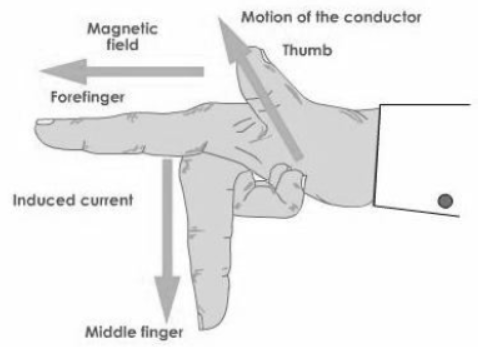INTEXT QUESTIONS PAGE NO. 231 AND 232

1. Which of the following property of a proton can change while it moves freely in a magnetic field? (There may be more than one correct answer.) (a) mass (b) speed (c) velocity (d) momentum
Answer:  Whenever a charged proton moves in a magnetic field, its velocity changes and as a result of this its momentum change. Thus (c) and (d) are the properties which change when a proton moves freely in a magnetic field.

2. In Activity 13.7, how do we think the displacement of rod AB will be affected if
(i) current in rod AB is increased;
(ii) a stronger horse-shoe magnet is used; and
(iii) length of the rod AB is increased?
(i) If the current in the rod AB is increased, force also increases.
(ii) When a stronger horse-shoe magnet is used, magnetic field increases as a result force also increases.
(iii)If the length of the rod AB is increased, force also increased.

3. A positively-charged particle (alpha-particle) projected towards west is deflected towards north by a magnetic field. The direction of magnetic field is (a) towards south (b) towards east (c) downward (d) upward
Answer: The direction of the motion of proton is the direction of current. The direction of force o the proton is towards north. Applying Fleming’s left hand rule, the direction of magnetic field is upward. The correct option is (d).

ELECTRIC MOTOR
An electric motor is a rotating device that converts electrical energy to mechanical energy. Electric motor is used as an important component in electric fans,
refrigerators, mixers, washing machines, computers, MP3 players etc.
Principle: When a coil carrying current is placed in a magnetic field, it experiences a torque. As a result of this torque, the coil begins to rotate.
Construction:
It consists of a rectangular coil ABCD of insulated copper wire. The coil is placed between the two poles of a magnetic field such that the arm AB and CD are perpendicular to the direction of the magnetic field. The ends of the coil are connected to the two halves P and Q of a split ring. The inner sides of these halves are insulated and attached to an axle. The external conducting edges of P and Q touch two conducting stationary brushes X and Y, respectively, as shown in the below figureWorking:
Current in the coil ABCD enters from the source battery through conducting brush X and flows back to the battery through brush Y.
Notice that the current in arm AB of the coil flows from A to B. In arm CD it flows from C to D, that is, opposite to the direction of current through arm AB.
On applying Fleming’s left hand rule for the direction of force on a current-carrying conductor in a magnetic field.. We find that the force acting on arm AB pushes it downwards while the force acting on arm CD pushes it upwards. Thus the coil and the axle O, mounted free to turn about an axis, rotate anti-clockwise.
At half rotation, Q makes contact with the brush X and P with brush Y. Therefore the current in the coil gets reversed and flows along the path DCBA. A device that reverses the direction of flow of current through a circuit is called a commutator. In electric motors, the split ring acts as a commutator.
The reversal of current also reverses the direction of force acting on the two arms AB and CD. Thus the arm AB of the coil that was earlier pushed down is now pushed up and the arm CD previously pushed up is now pushed down. Therefore the coil and the axle rotate half a turn more in the same direction. The reversing of the current is repeated at each half rotation, giving rise to a continuous rotation of the coil and to the axle.

Uses of electric motor:
The commercial motors use (i) an electromagnet in place of permanent magnet; (ii) large number of turns of the conducting wire in the current-carrying coil; and (iii) a soft iron core on which the coil is wound. The soft iron core, on which the coil is wound, plus the coils, is called an armature. This enhances the power of the motor.

INTEXT QUESTIONS PAGE NO. 231 AND 232

1. State Fleming’s left-hand rule.
Answer: Fleming’s left hand rule states that if we arrange the thumb, the centre finger, and the forefinger of the left hand at right angles to each other, then the thumb points towards the direction of the magnetic force, the centre finger gives the direction of current, and the forefinger points in the direction of magnetic field.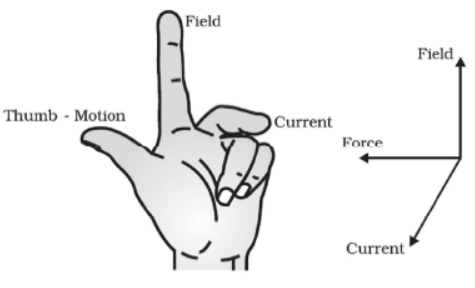2. What is the principle of an electric motor?
Answer: The working principle of an electric motor is based on the magnetic effect of current. A current-carrying loop experiences a force and rotates when placed in a magnetic field. The direction of rotation of the loop is given by the Fleming’s lefthand rule.

3. What is the role of the split ring in an electric motor?
Answer: The split ring in the electric motor acts as a commutator. The commutator reverses the direction of current flowing through the coil after each half rotation of the coil. Due to this reversal of the current, the coil continues to rotate in the same direction.

ELECTROMAGNETIC INDUCTION
The production of electricity from magnetism is called Electromagnetic induction.
When a straight wire is moved up and down rapidly between the poles of magnet, then an electric current is produced in the wire. This is an example of electromagnetic induction The process of electromagnetic induction has led to the construction of generators for producing electricity at power stations The current produced by moving a straight wire in a magnetic field is called an induced current. In the below figure, moving a magnet towards a coil sets up a current in the coil circuit, as indicated by deflection in the galvanometer needle.If the bar magnet moved towards south pole of the magnet towards the end B of the coil, the deflections in the galvanometer would just be opposite to the previous case.
When the coil and the magnet are both stationary, there is no deflection in the galvanometer. It is, thus, clear from this activity that motion of a magnet with respect to the coil produces an induced potential difference, which sets up an induced electric current in the circuit.

ELECTRIC GENERATOR
In an electric generator, mechanical energy is used to rotate a conductor in a magnetic field to produce electricity.
Principle: Whenever in a closed circuit, the magnetic field lines change, an induced current is produced.
Construction:
♦ An electric generator, as shown in the below figure, consists of a rotating rectangular coil ABCD placed between the two poles of a permanent magnet.
♦ The two ends of this coil are connected to the two rings R1 and R2.
♦ The inner side of these rings are made insulated.
♦ The two conducting stationary brushes B1 and B2 are kept pressed separately on the rings R1 and R2, respectively.
♦ The two rings R1 and R2 are internally attached to an axle.
♦ The axle may be mechanically rotated from outside to rotate the coil inside the magnetic field.
♦ Outer ends of the two brushes are connected to the galvanometer to show the flow of current in the given external circuit.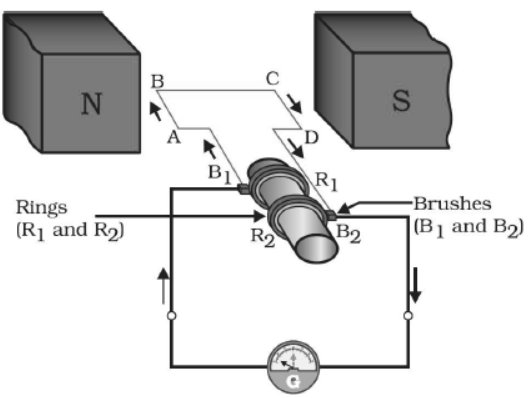Working:
When the axle attached to the two rings is rotated such that the arm AB moves up (and the arm CD moves down) in the magnetic field produced by the permanent magnet. Let us say the coil ABCD is rotated clockwise in the arrangement shown in the above figure.
By applying Fleming’s right-hand rule, the induced currents are set up in these arms along the directions AB and CD. Thus an induced current flows in the direction ABCD. If there are larger numbers of turns in the coil, the current generated in each turn adds up to give a large current through the coil. This means that the current in the external circuit flows from B2 to B1.

After half a rotation, arm CD starts moving up and AB moving down. As a result, the directions of the induced currents in both the arms change, giving rise to the net induced current in the direction DCBA. The current in the external circuit now flows from B1 to B2. Thus after every half rotation the polarity of the current in the respective arms changes. Such a current, which changes direction after equal intervals of time, is called an alternating current (abbreviated as AC). This device is called an AC generator.

To get a direct current (DC, which does not change its direction with time), a splitring type commutator must be used. With this arrangement, one brush is at all times in contact with the arm moving up in the field, while the other is in contact with the arm moving down. We have seen the working of a split ring commutator in the case of an electric motor Thus a unidirectional current is produced. The generator is thus called a DC generator. The difference between the direct and alternating currents is that the direct current always flows in one direction, whereas the alternating current reverses its direction periodically.

INTEXT QUESTIONS PAGE NO. 236

1. Explain different ways to induce current in a coil.
Answer: The different ways to induce current in a coil are as follows:
(a) If a coil is moved rapidly between the two poles of a horse-shoe magnet, then an electric current is induced in the coil.
(b) If a magnet is moved relative to a coil, then an electric current is induced in the coil

INTEXT QUESTIONS PAGE NO. 237

1. State the principle of an electric generator.
Answer: An electric generator works on the principle of electromagnetic induction. It generates electricity by rotating a coil in a magnetic field.

2. Name some sources of direct current.
Answer: Some sources of direct current are cell, DC generator, etc

3. Which sources produce alternating current?
Answer: AC generators, power plants, etc., produce alternating current

4. Choose the correct option: A rectangular coil of copper wires is rotated in a magnetic field. The direction of the induced current changes once in each
(a) two revolutions
(b) one revolution
(c) half revolution
(d) one-fourth revolution
Answer: (c) When a rectangular coil of copper is rotated in a magnetic field, the direction of the induced current in the coil changes once in each half revolution. As a result, the direction of current in the coil remains the same

DOMESTIC ELECTRIC CIRCUITS

When does an electric short circuit occur?
Answer: If the resistance of an electric circuit becomes very low, then the current flowing through the circuit becomes very high. This is caused by connecting too many appliances to a single socket or connecting high power rating appliances to the light circuits. This results in a short circuit.
When the insulation of live and neutral wires undergoes wear and tear and then touches each other, the current flowing in the circuit increases abruptly. Hence, a short circuit occurs.

What is the function of an earth wire? Why is it necessary to earth metallic appliances?
Answer: The metallic body of electric appliances is connected to the earth by means of earth wire so that any leakage of electric current is transferred to the ground. This prevents any electric shock to the user. That is why earthing of the electrical appliances is necessary.

What is Electric fuse? What is the important of electric fuse?
Answer: Electric Fuse consists of a piece of wire made of a metal or an alloy of appropriate melting point, for example aluminium, copper, iron, lead etc. If a current larger than the specified value flows through the circuit, the temperature of the fuse wire increases. This melts the fuse wire and breaks the circuit. Fuse is the most important safety device, used for protecting the circuits due to short-circuiting or overloading of the circuits. The use of an electric fuse prevents the electric circuit and the appliance from a possible damage by stopping the flow of unduly high electric current. The fuses used for domestic purposes are rated as 1 A, 2 A, 3 A, 5 A, 10 A, etc.

INTEXT QUESTIONS PAGE NO. 238

1. Name two safety measures commonly used in electric circuits and appliances.
Two safety measures commonly used in electric circuits and appliances are as follows:
Answer: (i) Each circuit must be connected with an electric fuse. This prevents the flow of excessive current through the circuit. When the current passing through the wire exceeds the maximum limit of the fuse element, the fuse melts to stop the flow of current through that circuit, hence protecting the appliances connected to the circuit.
(ii) Earthing is a must to prevent electric shocks. Any leakage of current in an electric appliance is transferred to the ground and people using the appliance do not get the shock.

2. An electric oven of 2 kW power rating is operated in a domestic electric circuit (220 V) that has a current rating of 5 A. What result do you expect? Explain.
Answer: Current drawn by the electric oven can be obtained by the expression,
P=VI
I = P/V
Where, current = I. Power of the oven, P = 2 kW = 2000W
Voltage supplied, V = 220V
I = 2000/220 = 9.09 A
Hence, the current drawn by the electric oven is 9.09 A, which exceeds the safe limit of the circuit. Fuse element of the electric fuse will melt and break the circuit.

3. What precaution should be taken to avoid the overloading of domestic electric circuits?
Answer: The precautions that should be taken to avoid the overloading of domestic circuits are as follows:
(a) Too many appliances should not be connected to a single socket.
(b) Too many appliances should not be used at the same time.
(c) Faulty appliances should not be connected in the circuit
(d) Fuse should be connected in the circuit.

MAGNETISM IN MEDICINE
An electric current always produces a magnetic field. Even weak ion currents that travel along the nerve cells in our body produce magnetic fields. When we touch something, our nerves carry an electric impulse to the muscles we need to use. This impulse produces a temporary magnetic field. These fields are very weak and are about one-billionth of the earth’s magnetic field. Two main organs in the human body where the magnetic field produced is significant, are the heart and the brain. The magnetic field inside the body forms the basis of obtaining the images of different body parts. This is done using a technique called Magnetic Resonance Imaging (MRI). Analysis of these images helps in medical diagnosis. Magnetism has, thus, got important uses in medicine.

EXERCISE QUESTIONS PAGE NO. 240

1. Which of the following correctly describes the magnetic field near a long straight wire?
(a) The field consists of straight lines perpendicular to the wire
(b) The field consists of straight lines parallel to the wire
(c) The field consists of radial lines originating from the wire
(d) The field consists of concentric circles centred on the wire

2. The phenomenon of electromagnetic induction is
(a) the process of charging a body
(b) the process of generating magnetic field due to a current passing through a coil
(c) producing induced current in a coil due to relative motion between a magnet and the coil
(d) the process of rotating a coil of an electric motor

3. The device used for producing electric current is called a
(a) generator.
(b) galvanometer.
(c) ammeter.
(d) motor.

4. The essential difference between an AC generator and a DC generator is that
(a) AC generator has an electromagnet while a DC generator has permanent magnet.
(b) DC generator will generate a higher voltage.
(c) AC generator will generate a higher voltage.
(d) AC generator has slip rings while the DC generator has a commutator.

5. At the time of short circuit, the current in the circuit
(a) reduces substantially.
(b) does not change.
(c) increases heavily.
(d) vary continuously.

6. State whether the following statements are true or false.
(a) An electric motor converts mechanical energy into electrical energy.
(b) An electric generator works on the principle of electromagnetic induction.
(c) The field at the centre of a long circular coil carrying current will be parallel straight lines.
(d) A wire with a green insulation is usually the live wire of an electric supply.

7. List three sources of magnetic fields.
Answer: Three sources of magnetic fields are as follows:
(a) Current-carrying conductors
(b) Permanent magnets
(c) Electromagnets

8. How does a solenoid behave like a magnet? Can you determine the north and south poles of a current–carrying solenoid with the help of a bar magnet? Explain.
Answer: A solenoid is a long coil of circular loops of insulated copper wire. Magnetic field lines are produced around the solenoid when a current is allowed to flow through it. The magnetic field produced by it is similar to the magnetic field of a bar magnet. The field lines produced in a current-carrying solenoid is shown in the following figure.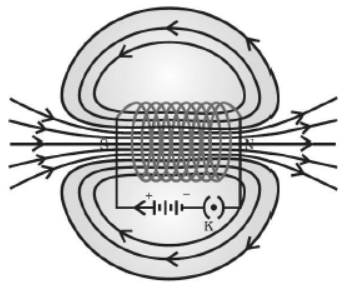In the above figure, when the north pole of a bar magnet is brought near the end connected to the negative terminal of the battery, the solenoid repels the bar magnet. Since like poles repel each other, the end connected to the negative terminal of the battery behaves as the north pole of the solenoid and the other end behaves as a south pole. Hence, one end of the solenoid behaves as a north pole and the other end behaves as a south pole.

9. When is the force experienced by a current–carrying conductor placed in a magnetic field largest?
Answer: The force experienced by a current-currying conductor is the maximum when the direction of current is perpendicular to the direction of the magnetic field.

10. Imagine that you are sitting in a chamber with your back to one wall. An electron beam, moving horizontally from back wall towards the front wall, is deflected by a strong magnetic field to your right side. What is the direction of magnetic field?
Answer: The direction of magnetic field is given by Fleming’s left hand rule.
Magnetic field inside the chamber will be perpendicular to the direction of current (opposite to the direction of electron) and direction of deflection/force i.e., either upward or downward. The direction of current is from the front wall to the back wall because negatively charged electrons are moving from back wall to the front wall. The direction of magnetic force is rightward. Hence, using Fleming’s left hand rule, it can be concluded that the direction of magnetic field inside the chamber is downward.

11. Draw a labelled diagram of an electric motor. Explain its principle and working.
What is the function of a split ring in an electric motor?
Answer: An electric motor is a rotating device that converts electrical energy to mechanical energy. Electric motor is used as an important component in electric fans, refrigerators, mixers, washing machines, computers, MP3 players etc.
Principle: When a coil carrying current is placed in a magnetic field, it experiences a torque. As a result of this torque, the coil begins to rotate.

Construction:
It consists of a rectangular coil ABCD of insulated copper wire. The coil is placed between the two poles of a magnetic field such that the arm AB and CD are perpendicular to the direction of the magnetic field. The ends of the coil are connected to the two halves P and Q of a split ring. The inner sides of these halves are insulated and attached to an axle. The external conducting edges of P and Q touch two conducting stationary brushes X and Y, respectively, as shown in the below figureWorking:
Current in the coil ABCD enters from the source battery through conducting brush X and flows back to the battery through brush Y.
Notice that the current in arm AB of the coil flows from A to B. In arm CD it flows from C to D, that is, opposite to the direction of current through arm AB.
On applying Fleming’s left hand rule for the direction of force on a currentcarrying conductor in a magnetic field.. We find that the force acting on arm AB pushes it downwards while the force acting on arm CD pushes it upwards. Thus the coil and the axle O, mounted free to turn about an axis, rotate anti-clockwise.
At half rotation, Q makes contact with the brush X and P with brush Y. Therefore the current in the coil gets reversed and flows along the path DCBA. A device that reverses the direction of flow of current through a circuit is called a commutator.
In electric motors, the split ring acts as a commutator.
The reversal of current also reverses the direction of force acting on the two arms AB and CD. Thus the arm AB of the coil that was earlier pushed down is now pushed up and the arm CD previously pushed up is now pushed down. Therefore the coil and the axle rotate half a turn more in the same direction. The reversing of the current is repeated at each half rotation, giving rise to a continuous rotation of the coil and to the axle.

12. Name some devices in which electric motors are used.
Answer: Some devices in which electric motors are used are as follows:
(a) Water pumps (b) Electric fans (c) Electric mixers (d) Washing machines

13. A coil of insulated copper wire is connected to a galvanometer. What will happen if a bar magnet is (i) pushed into the coil, (ii) withdrawn from inside the coil, (iii) held stationary inside the coil?
Answer: A current induces in a solenoid if a bar magnet is moved relative to it. This is the principle of electromagnetic induction.
(i) When a bar magnet is pushed into a coil of insulated copper wire, a current is induced momentarily in the coil. As a result, the needle of the galvanometer deflects momentarily in a particular direction.
(ii) When the bar magnet is withdrawn from inside the coil of the insulated copper wire, a current is again induced momentarily in the coil in the opposite direction.
As a result, the needle of the galvanometer deflects momentarily in the opposite direction.
(iii) When a bar magnet is held stationary inside the coil, no current will be induced in the coil. Hence, galvanometer will show no deflection.

14. Two circular coils A and B are placed closed to each other. If the current in the coil A is changed, will some current be induced in the coil B? Give reason.
Answer: Two circular coils A and B are placed closed to each other. When the current in coil A is changed, the magnetic field associated with it also changes. As a result, the magnetic field around coil B also changes. This change in magnetic field lines around coil B induces an electric current in it. This is called electromagnetic induction.

15. State the rule to determine the direction of a (i) magnetic field produced around a straight conductor-carrying current, (ii) force experienced by a current-carrying straight conductor placed in a magnetic field which is perpendicular to it, and (iii) current induced in a coil due to its rotation in a magnetic field.
Answer: (i) Maxwell’s right hand thumb rule
(ii) Fleming’s left hand rule
(iii) Fleming’s right hand rule

16. Explain the underlying principle and working of an electric generator by drawing a labelled diagram. What is the function of brushes?
Answer: In an electric generator, mechanical energy is used to rotate a conductor in a magnetic field to produce electricity.
Principle: Whenever in a closed circuit, the magnetic field lines change, an induced current is produced.
Construction:
♦ An electric generator, as shown in the below figure, consists of a rotating rectangular coil ABCD placed between the two poles of a permanent magnet.
♦ The two ends of this coil are connected to the two rings R1 and R2.
♦ The inner side of these rings are made insulated.
♦ The two conducting stationary brushes B1 and B2 are kept pressed separately on the rings R1 and R2, respectively.
♦ The two rings R1 and R2 are internally attached to an axle.
♦ The axle may be mechanically rotated from outside to rotate the coil inside the magnetic field.
♦ Outer ends of the two brushes are connected to the galvanometer to show the flow of current in the given external circuit.
Working:
When the axle attached to the two rings is rotated such that the arm AB moves up (and the arm CD moves down) in the magnetic field produced by the permanent magnet. Let us say the coil ABCD is rotated clockwise in the arrangement shown in the above figure.
By applying Fleming’s right-hand rule, the induced currents are set up in these arms along the directions AB and CD. Thus an induced current flows in the direction ABCD. If there are larger numbers of turns in the coil, the current generated in each turn adds up to give a large current through the coil. This means that the current in the external circuit flows from B2 to B1.

After half a rotation, arm CD starts moving up and AB moving down. As a result, the directions of the induced currents in both the arms change, giving rise to the net induced current in the direction DCBA. The current in the external circuit now flows from B1 to B2. Thus after every half rotation the polarity of the current in the respective arms changes. Such a current, which changes direction after equal intervals of time, is called an alternating current (abbreviated as AC). This device is called an AC generator.

To get a direct current (DC, which does not change its direction with time), a split-ring type commutator must be used. With this arrangement, one brush is at all times in contact with the arm moving up in the field, while the other is in contact with the arm moving down. We have seen the working of a split ring commutator in the case of an electric motor Thus a unidirectional current is produced. The generator is thus called a DC generator. The difference between the direct and alternating currents is that the direct current always flows in one direction, whereas the alternating current reverses its direction periodically.

17. When does an electric short circuit occur?
Answer: If the resistance of an electric circuit becomes very low, then the current flowing through the circuit becomes very high. This is caused by connecting too many appliances to a single socket or connecting high power rating appliances to the light circuits. This results in a short circuit.
When the insulation of live and neutral wires undergoes wear and tear and then touches each other, the current flowing in the circuit increases abruptly. Hence, a short circuit occurs.

18. What is the function of an earth wire? Why is it necessary to earth metallic appliances?
Answer: The metallic body of electric appliances is connected to the earth by means of earth wire so that any leakage of electric current is transferred to the ground.
This prevents any electric shock to the user. That is why earthing of the electrical appliances is necessary.

Refer to attached file for CBSE Class 10 Science Magnetic Effects of Electric Current Assignment Set C

 CBSE Class 10 Science Chemical Reactions and Equations Assignment
 CBSE Class 10 Science Acids Bases and Salts Assignment Set A CBSE Class 10 Science Acids Bases and Salts Assignment Set B CBSE Class 10 Science Acids Bases and Salts Assignment Set C
 CBSE Class 10 Science Metals and Non Metals Assignment Set A CBSE Class 10 Science Metals and Non Metals Assignment Set B
 CBSE Class 10 Science Carbon and its compounds Assignment Set A CBSE Class 10 Science Carbon and its compounds Assignment Set B CBSE Class 10 Science Carbon and its compounds Assignment Set C CBSE Class 10 Science Carbon and its compounds Assignment Set D CBSE Class 10 Science Carbon and its compounds Assignment Set E
 CBSE Class 10 Science Periodic Classification Of Elements Assignment Set A CBSE Class 10 Science Periodic Classification Of Elements Assignment Set B CBSE Class 10 Science Periodic Classification Of Elements Assignment Set C
 CBSE Class 10 Science Biology Life Process Respiration Assignment CBSE Class 10 Science Biology Nutrition Assignment CBSE Class 10 Science Biology Respiration Assignment CBSE Class 10 Science Biology Transport Enrichment Assignment CBSE Class 10 Science Biology Transportation Assignment CBSE Class 10 Science Life Processes Assignment Set A CBSE Class 10 Science Life Processes Assignment Set B CBSE Class 10 Science Life Processes Assignment Set C
 CBSE Class 10 Science Control and Coordination Assignment Set A CBSE Class 10 Science Control and Coordination Assignment Set B CBSE Class 10 Science Control and Coordination Assignment Set C CBSE Class 10 Science Control and Coordination Assignment Set D
 CBSE Class 10 Science How do Organisms Reproduce Assignment Set A CBSE Class 10 Science How do Organisms Reproduce Assignment Set B
 CBSE Class 10 Science Heredity and Evolution Assignment Set A CBSE Class 10 Science Heredity and Evolution Assignment Set B CBSE Class 10 Science Heredity and Evolution Assignment Set C
 CBSE Class 10 Science Light Reflection and Refraction Assignment Set A CBSE Class 10 Science Light Reflection and Refraction Assignment Set B CBSE Class 10 Science Light Reflection and Refraction Assignment Set C CBSE Class 10 Science Light Reflection and Refraction Assignment Set D CBSE Class 10 Science Light Reflection and Refraction Assignment Set E CBSE Class 10 Science Light Reflection and Refraction Assignment Set F
 CBSE Class 10 Science Human Eye and Colorful World Assignment Set A CBSE Class 10 Science Human Eye and Colorful World Assignment Set B CBSE Class 10 Science Human Eye and Colorful World Assignment Set C
 CBSE Class 10 Physics Electricity Assignment Set A CBSE Class 10 Physics Electricity Assignment Set B CBSE Class 10 Physics Electricity Assignment Set C CBSE Class 10 Science Electricity Assignment Set A CBSE Class 10 Science Electricity Assignment Set B CBSE Class 10 Science Electricity Assignment Set C
 CBSE Class 10 Science Magnetic Effects of Electric Current Assignment Set A CBSE Class 10 Science Magnetic Effects of Electric Current Assignment Set B CBSE Class 10 Science Magnetic Effects of Electric Current Assignment Set C CBSE Class 10 Science Magnetic Effects of Electric Current Assignment Set D
 CBSE Class 10 Science Sources of Energy Assignment Set A CBSE Class 10 Science Sources of Energy Assignment Set B CBSE Class 10 Science Sources of Energy Assignment Set C CBSE Class 10 Science Sources of Energy Assignment Set D
 CBSE Class 10 Science Our Environment Assignment Set A CBSE Class 10 Science Our Environment Assignment Set B CBSE Class 10 Science Our Environment Assignment Set C
 CBSE Class 10 Science Sustainable Management of Natural Resources Assignment Set A CBSE Class 10 Science Sustainable Management of Natural Resources Assignment Set B CBSE Class 10 Science Sustainable Management of Natural Resources Assignment Set C
 CBSE Class 10 Physics Revision Assignment Set A CBSE Class 10 Physics Revision Assignment Set B CBSE Class 10 Physics Revision Assignment Set C CBSE Class 10 Physics Revision Assignment Set D CBSE Class 10 Physics Revision Assignment Set E CBSE Class 10 Physics Revision Assignment Set F CBSE Class 10 Physics Revision Assignment Set G CBSE Class 10 Physics Revision Assignment Set H
 CBSE Class 10 Science Assignment CBSE Class 10 Science Assignments Collection CBSE Class 10 Science Energy Crossword Puzzle Assignment
 CBSE Class 10 Physics Question Bank CBSE Class 10 Physics Tungsten Assignment
 CBSE Class 10 Science Chemistry Cleaning Capacity of Soap Assignment CBSE Class 10 Science Chemistry Determination of pH Assignment CBSE Class 10 Science Chemistry Different Types of Chemical Reactions Assignment CBSE Class 10 Science Chemistry Displacement Reaction and Reactivity Series Assignment CBSE Class 10 Science Chemistry Properties of Acetic Acid Assignment CBSE Class 10 Science Chemistry Saponification Preparation of Soap Assignment CBSE Class 10 Science Chemistry Study of properties of HCI Acid and NaOH Assignment

Tags